#### Obstacle region for a rigid body

Suppose that the world,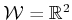or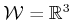, contains an obstacle region,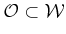. Assume here that a rigid robot,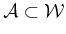, is defined; the case of multiple links will be handled shortly. Assume that both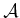and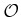are expressed as semi-algebraic models (which includes polygonal and polyhedral models) from Section 3.1. Let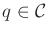denote the configuration of, in which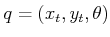forandfor(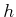represents the unit quaternion).

The obstacle region,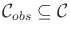, is defined as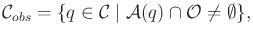(4.34)

which is the set of all configurations,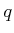, at which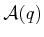, the transformed robot, intersects the obstacle region,. Sinceandare closed sets in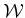, the obstacle region is a closed set in.

The leftover configurations are called the free space, which is defined and denoted as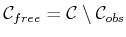. Sinceis a topological space and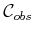is closed,must be an open set. This implies that the robot can come arbitrarily close to the obstacles while remaining in. Iftouches'',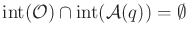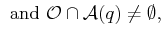(4.35)

then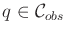(recall that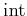means the interior). The condition above indicates that only their boundaries intersect.

The idea of getting arbitrarily close may be nonsense in practical robotics, but it makes a clean formulation of the motion planning problem. Sinceis open, it becomes impossible to formulate some optimization problems, such as finding the shortest path. In this case, the closure,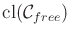, should instead be used, as described in Section 7.7.

Steven M LaValle 2020-08-14